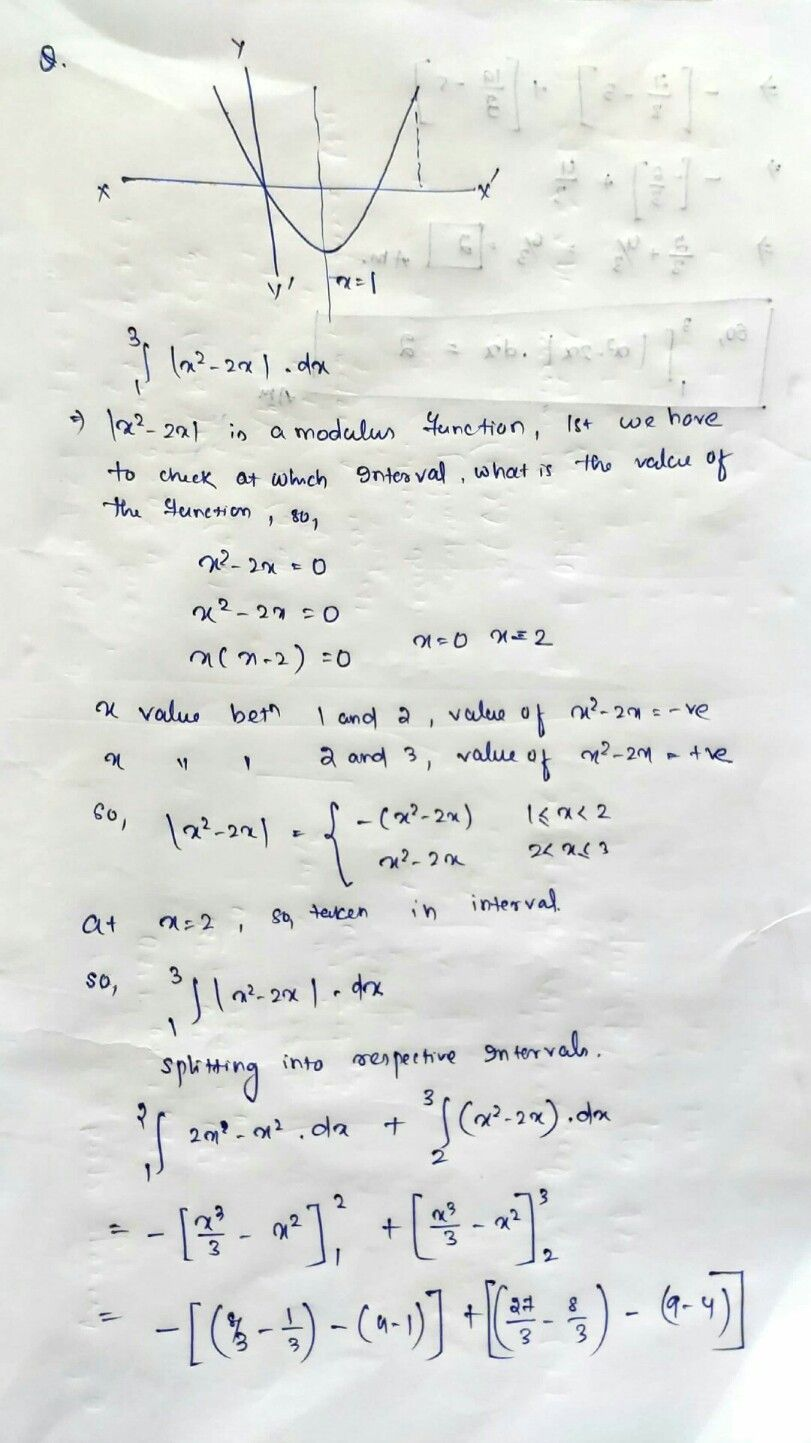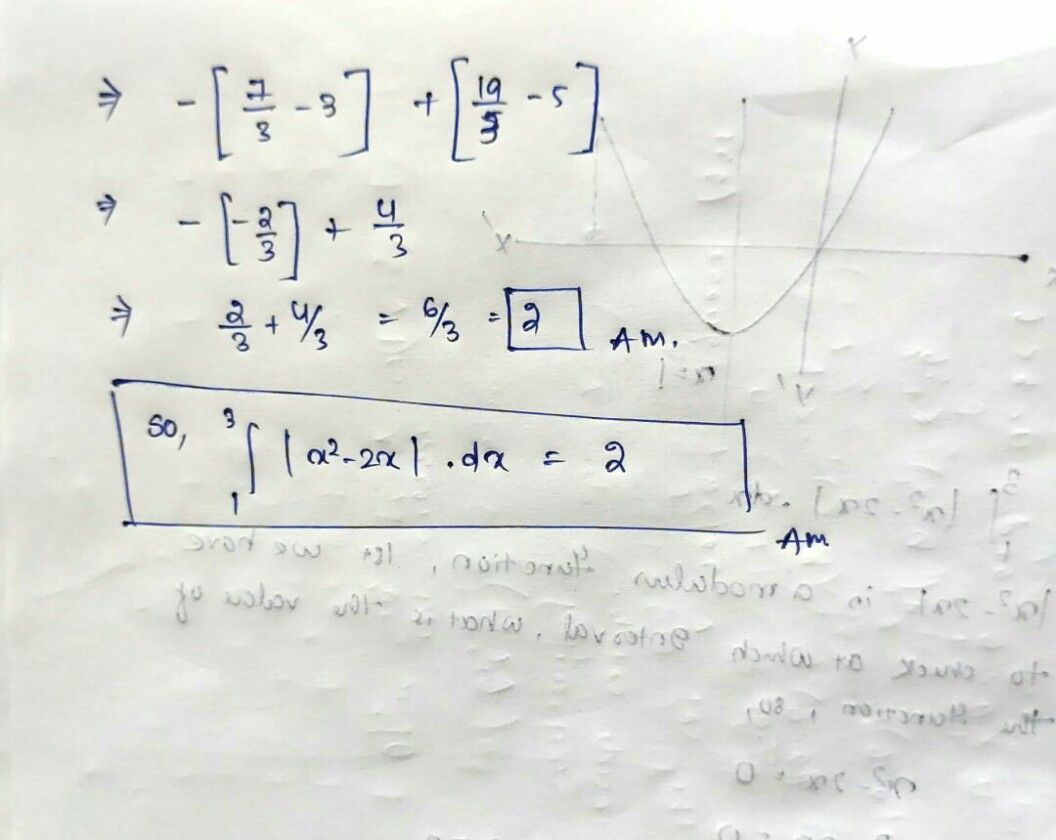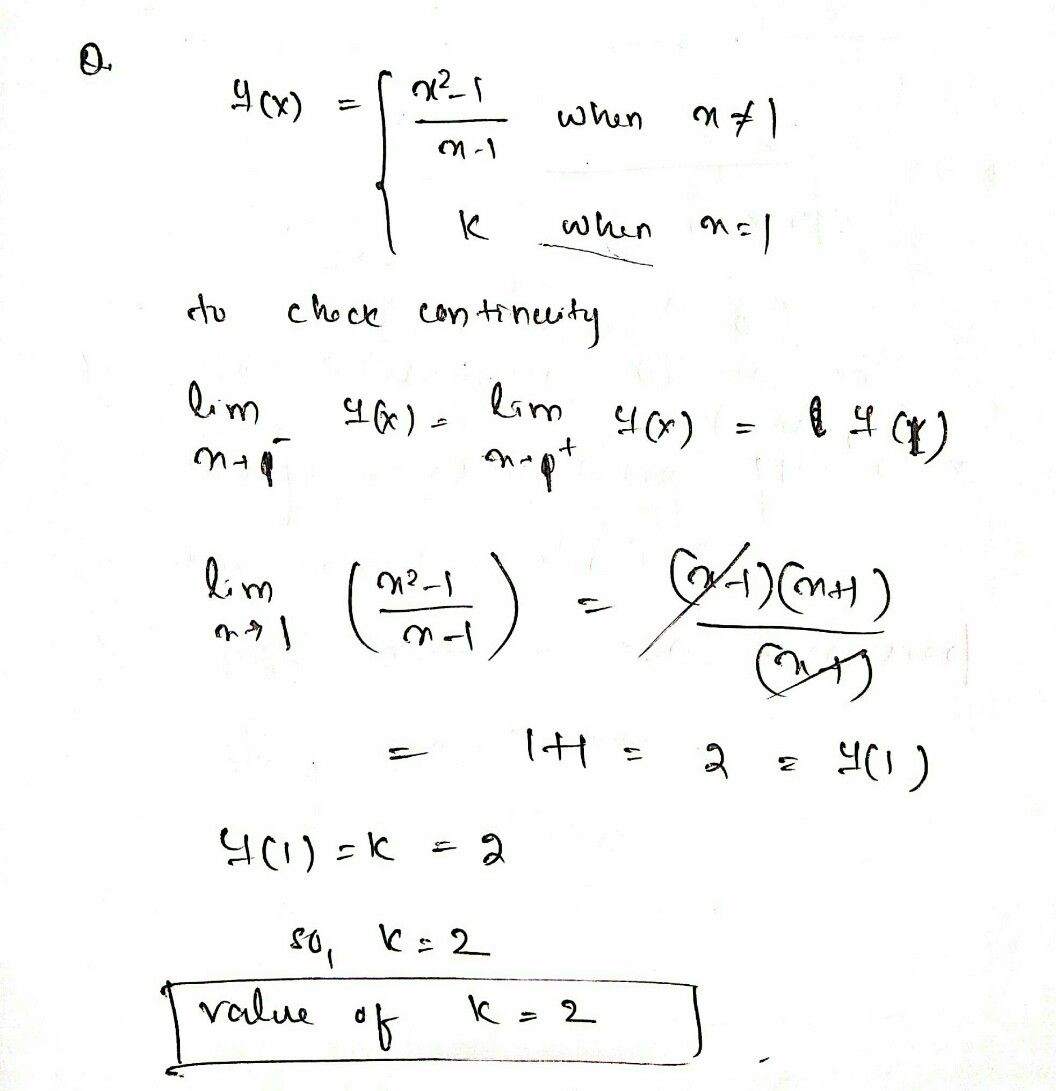Symbol
Problem$2.$ Evaluate $\int ^{3}|x^{2}-2x|dx$ $1$ $3.$ when $x≠1$ If the function $f\left(x\right)= \begin{cases} \dfrac {x^{2}-1} {x-1} \\ k \end{cases}$ when $x=1$ is given to be continuous at $x=1,$ then the value of $k$ $1S$